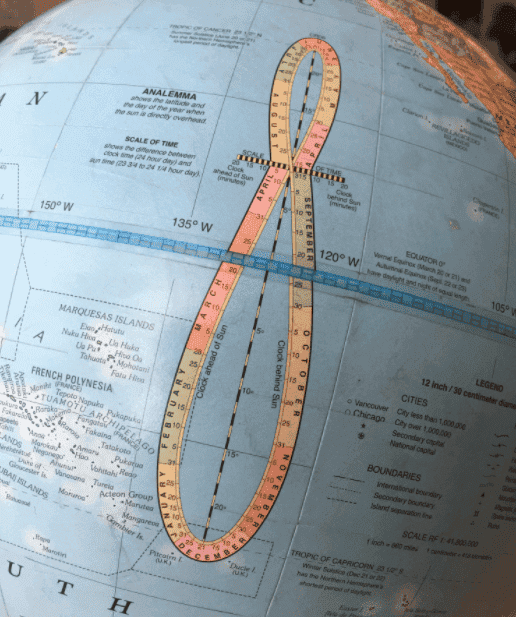# Does an Analemma visualize the Earth's actual orbit around the sun?

• Stargazing
• lolsurround
In summary, the analemma is a shape formed by taking a picture of the sun at the same time each day, showing the Earth's elliptical orbit and rotation. It is not the "real" orbit of Earth around the sun, but rather a representation of the equation of time and declination of the sun. It has limited practical uses, such as decorating a globe or using it as a tool for position finding.

#### lolsurround

An Analemma is the shape that arises from taking a picture of the sun everyday at the same time of the day. This shape also have depth to it since Earth's orbit is elliptical, showing what looks like an wobbly orbit. Isn't this the "real" orbit of Earth around the sun?

lolsurround said:
An Analemma is the shape that arises from taking a picture of the sun everyday at the same time of the day. This shape also have depth to it since Earth's orbit is elliptical, showing what looks like an wobbly orbit. Isn't this the "real" orbit of Earth around the sun?
I love analemmas.

But the analemma reflects both the Earth's orbit around the Sun and the Earth's rotation on it's own axis. The combined effect gives us the equation of time. https://en.wikipedia.org/wiki/Equation_of_time
The equation of time is the east or west component of the analemma, a curve representing the angular offset of the Sun from its mean position on the celestial sphere as viewed from Earth. The equation of time values for each day of the year, compiled by astronomical observatories, were widely listed in almanacs and ephemerides.
So the answer to "Isn't this the "real" orbit of Earth around the sun?" is no because we are on the surface of Earth, not the center of Earth.

••BillTre and berkeman
anorlunda said:
But the analemma reflects both the Earth's orbit around the Sun and the Earth's rotation on it's own axis
The analemma is different from year to year. A particular time on a particular day of the year changes its position on that 'figure of eight'. If we didn't use leap years / leap seconds etc. etc. that place would march around and around the '8' every year. That would only be good for some solar astronomers. Catching the train on time could not be relied on.

Good point. You can't use it as a clock or a sundial. Actually, I can't think of a practical use for analemmas other than decorating a world globe and creating beautiful pictures. Proving your latitude without use of a sextant is the most far fetched application I can think of.•sophiecentaur
The analemma shows the Equation of Time and the Declination of the Sun through the year. It can be considered as portraying the slow movement of the Sun's Geographical position on the Earth's surface each day. By knowing the Sun's GP and by measuring its altitude with a sextant, we can draw a circle passing through our (unknown) position. By repeating this measurement a few hours later we can obtain another circle, and where they cross is our position. This is the basic principle of position finding when not at noon.